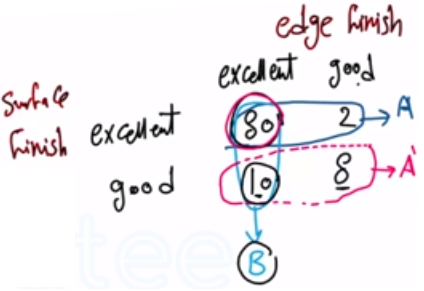Need Help?

Subscribe to Probability

###### \${selected_topic_name}
• Notes

Samples of a cast aluminum part are classified on the
basis of surface finish (in microinches) and edge finish. The
results of 100 parts are summarized as follows:

$\begin{array}{cccc}{} & {} & {\frac{\text { edge finish }}{\text { excellent }}} & {\text { good }} \\ {\text { surface }} & {\text { excellent }} & {80} & {2} \\ {\text { finish }} & {\text { good }} & {10} & {8}\end{array}$

Let $A$ denote the event that a sample has excellent surface fin-
ish, and let $B$ denote the event that a sample has excellent
length. If a part is selected at random, determine the following
probabilities:

$\begin{array}{ll}{\text { (a) } P(A)} & {\text { (b) } P(B)} \\ {\text { (c) } P\left(A^{\prime}\right)} & {\text { (d) } P(A \cap B)} \\ {\text { (e) } P(A \cup B)} & {\text { (f) } P\left(A^{\prime} \cup B\right)}\end{array}$$A \rightarrow$ excellent surface finish

$B \rightarrow$ excellent edge finish

a) $P(A) \rightarrow P(A)=\frac{n(A)}{n(S)}=\frac{80+2}{100}=0.82$

b) $P(B) \rightarrow P(B)=\frac{n(B)}{n(S)}=\frac{80+10}{100}=0.90$

c) $P\left(A^{\prime}\right) \rightarrow P\left(A^{\prime}\right)=1-P(A)=1-0.82=0.18$

d) $P(A \cap B) \rightarrow P(A \cap B)=\frac{80}{100}=0.80$

e) $P(A \cup B) \rightarrow P(A \cup B)=\frac{2+80+10}{100}=0.92$

f) $P\left(A^{\prime} \cup B\right)$

$P\left(A^{\prime} \cup B\right)=\frac{8+10+80}{100}=0.98$